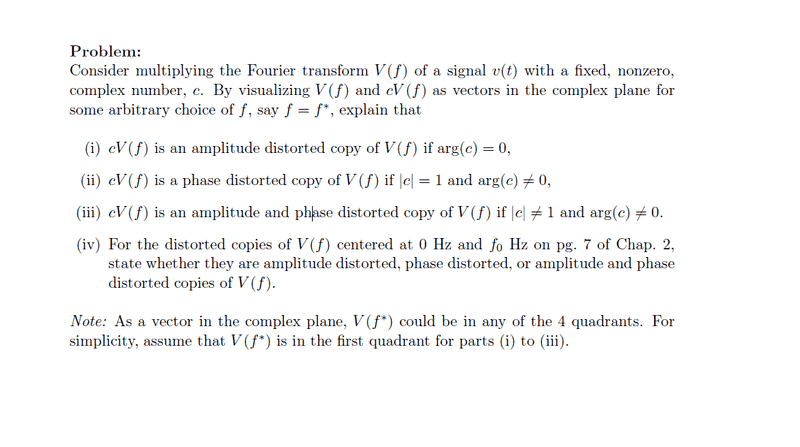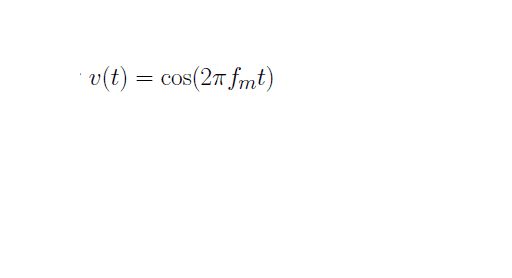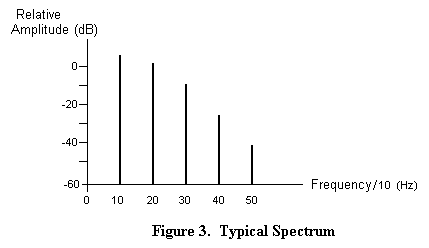# Linking Fourier Transform, Vectors and Complex Numbers

galaxy_twirl

## Homework Statement## The Attempt at a Solution

I tried to attempt the question but I am not sure how to start it, at least for part (i).

My biggest question, I think, is how does the multiplication of a random complex number to a Fourier-Transformed signal (V(f)) have an effect on V(f)? Although the question did not specify the type of signal that has been Fourier-Transformed, I shall assume that the original signal is a cosine waveform, as shown below:with fm=1.

In the question, it also mentioned to visualise V(f) and cV(f) as vectors in the complex plane, but how should I do it? Are there any diagrams online that show me how to represent cV(f) and V(f) as vectors in the complex plane?

Thank you. :)

milesyoung
I'd suggest you start by answering the following:
1. For some frequency ##f##, what does the modulus and argument of ##V(f)## represent? The first paragraph of the Wiki should be helpful.
2. If you have two complex numbers in polar form ##z_1 = r_1\angle\phi_1,z_2 = r_2\angle\phi_2##, what is their product ##z_1 z_2## equal to?

galaxy_twirl
I'd suggest you start by answering the following:
1. For some frequency ##f##, what does the modulus and argument of ##V(f)## represent? The first paragraph of the Wiki should be helpful.
2. If you have two complex numbers in polar form ##z_1 = r_1\angle\phi_1,z_2 = r_2\angle\phi_2##, what is their product ##z_1 z_2## equal to?

1. The modulus of ##V(f)## represents the amplitude of ##v(t)##, while the argument of ##V(f)## represents the phase angle of ##v(f)##.

2. The product should be equals to ##r_1r_2\angle(\phi_1+\phi_2)##. Am I right to say this?

Thank you. :)

milesyoung
1. The modulus of ##V(f)## represents the amplitude of ##v(t)##, while the argument of ##V(f)## represents the phase angle of ##v(f)##.
The modulus and argument of ##V(f)## represents the amplitude and phase, respectively, of the frequency component at ##f##, but you get the idea.

2. The product should be equals to ##r_1r_2\angle(\phi_1+\phi_2)##. Am I right to say this?
Yes, you are. For your first question (i), you again have two complex numbers ##c## and ##V(f)##, so what can you say about their product ##cV(f)##?

galaxy_twirl
Yes, you are. For your first question (i), you again have two complex numbers ##c## and ##V(f)##, so what can you say about their product ##cV(f)##?

There will be an increase in the amplitude of the wave which has been Fourier Transformed, while for the angle, it would be shifted x radians away to the left, since the angles will add up when ##c## is multiplied to ##V(f)##. Am I right to say this? :)

milesyoung
There will be an increase in the amplitude of the wave which has been Fourier Transformed, while for the angle, it would be shifted x radians away to the left, since the angles will add up when ##c## is multiplied to ##V(f)##. Am I right to say this? :)
You're talking about the vector interpretation of complex numbers? Like, if ##\arg(c)## is positive, the vector ##V(f)## would be scaled and rotated counterclockwise in the complex plane? That's how I understand what you wrote, and it's true, so it seems you already know everything you need to solve your assignment.

Do you have any questions?

galaxy_twirl
I was visualising it from a mathematical viewpoint, as in the earlier question, multiplying 2 complex numbers, ##z_1## and ##z_2##.

Right now, I still can't really link the vector diagram of complex numbers to the plot of amplitude-frequency of waves, I think. >< The latter, from my point of view, is a diagram of spikes coming out at different frequencies, depending on the original wave.

I do agree that the vector ##V(f)## will be rotated anti-clockwise as the summing of angles is positive.

Thanks a lot for your help! :D

milesyoung
Right now, I still can't really link the vector diagram of complex numbers to the plot of amplitude-frequency of waves, I think. >< The latter, from my point of view, is a diagram of spikes coming out at different frequencies, depending on the original wave.
Ah, you mean the amplitude spectrum, e.g:But that's just a plot of ##|V(f)|## over some interval of ##f##, i.e. it's a plot of the modulus of ##V(f)##. In some contexts, you also include the phase spectrum, which is just a plot of ##\arg(V(f))##.

Does that help?

galaxy_twirl
Ah, you mean the amplitude spectrum, e.g:But that's just a plot of ##|V(f)|## over some interval of ##f##, i.e. it's a plot of the modulus of ##V(f)##. In some contexts, you also include the phase spectrum, which is just a plot of ##\arg(V(f))##.

Does that help?

I see. Yupp, I meant that. Haha. That was the diagram shown in my lecture notes, so I can link to it better. :)

Yes, and thank you so much for your help! :D I should be fine with my assignment now.